1. /
2. CBSE
3. /
4. Class 07
5. /
6. Mathematics
7. /
8. NCERT Solutions for Class...

# NCERT Solutions for Class 7 Maths Exercise 2.2### myCBSEguide App

Download the app to get CBSE Sample Papers 2023-24, NCERT Solutions (Revised), Most Important Questions, Previous Year Question Bank, Mock Tests, and Detailed Notes.

NCERT solutions for Maths Fractions and Decimals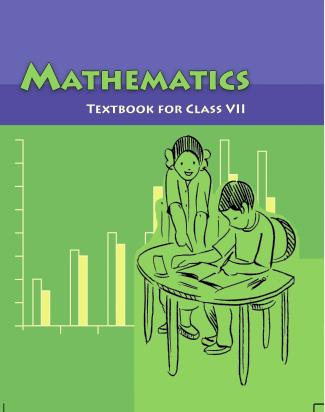## NCERT Solutions for Class 7 Maths Fractions and Decimals

###### Question 1.Which of the drawings {tex}\left( a \right){/tex} to {tex}\left( d \right){/tex} show:

(i){tex}2 \times \frac{1}{5}{/tex}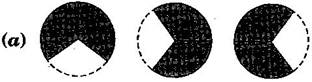(ii){tex}2 \times \frac{1}{2}{/tex}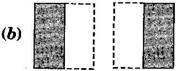(iii){tex}3 \times \frac{2}{3}{/tex}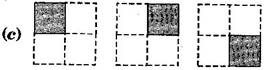(iv){tex}3 \times \frac{1}{4}{/tex}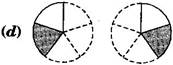(i) – (d) Since {tex}2 \times \frac{1}{5} = \frac{1}{5} + \frac{1}{5}{/tex}

(ii) – (b) Since {tex}2 \times \frac{1}{2} = \frac{1}{2} + \frac{1}{2}{/tex}

(iii) – (a) Since {tex}3 \times \frac{2}{3} = \frac{2}{3} + \frac{2}{3} + \frac{2}{3}{/tex}

(iv) – (c) Since {tex}3 \times \frac{1}{4} = \frac{1}{4} + \frac{1}{4} + \frac{1}{4}{/tex}

NCERT Solutions for Class 7 Maths Exercise 2.2

###### Question 2.Some pictures {tex}\left( a \right){/tex} to {tex}\left( c \right){/tex} are given below. Tell which of them show:

(i){tex}3 \times \frac{1}{5} = \frac{3}{5}{/tex}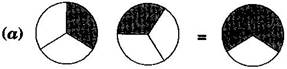(ii){tex}2 \times \frac{1}{3} = \frac{2}{3}{/tex}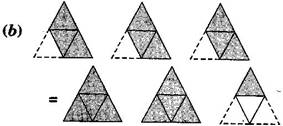(iii){tex}3 \times \frac{3}{4} = 2\frac{1}{4}{/tex}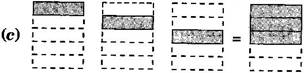(i) – (c) Since {tex}3 \times \frac{1}{5} = \frac{1}{5} + \frac{1}{5} + \frac{1}{5}{/tex}

(ii) – (a) Since {tex}2 \times \frac{1}{3} = \frac{1}{3} + \frac{1}{3}{/tex}

(iii) – (b) Since {tex}3 \times \frac{3}{4} = \frac{3}{4} + \frac{3}{4} + \frac{3}{4}{/tex}

NCERT Solutions for Class 7 Maths Exercise 2.2

###### Question 3.Multiply and reduce to lowest form and convert into a mixed fraction:

(i) {tex}7 \times \frac{3}{5}{/tex}

(ii) {tex}4 \times \frac{1}{3}{/tex}

(iii) {tex}2 \times \frac{6}{7}{/tex}

(iv) {tex}5 \times \frac{2}{9}{/tex}

(v) {tex}\frac{2}{3} \times 4{/tex}

(vi) {tex}\frac{5}{2} \times 6{/tex}

(vii) {tex}11 \times \frac{4}{7}{/tex}

(viii) {tex}20 \times \frac{4}{5}{/tex}

(ix) {tex}13 \times \frac{1}{3}{/tex}

(x) {tex}15 \times \frac{3}{5}{/tex}

(i) {tex}7 \times \frac{3}{5}{/tex} = {tex}\frac{{7 \times 3}}{5}{/tex} = {tex}\frac{{21}}{5} = 4\frac{1}{5}{/tex}

(ii) {tex}4 \times \frac{1}{3}{/tex} = {tex}\frac{{4 \times 1}}{3}{/tex} = {tex}\frac{4}{3} = 1\frac{1}{3}{/tex}

(iii){tex}2 \times \frac{6}{7}{/tex} = {tex}\frac{{2 \times 6}}{7}{/tex} = {tex}\frac{{12}}{7} = 1\frac{5}{7}{/tex}

(iv) {tex}5 \times \frac{2}{9}{/tex} = {tex}\frac{{5 \times 2}}{9}{/tex} = {tex}\frac{{10}}{9} = 1\frac{1}{9}{/tex}

(v) {tex}\frac{2}{3} \times 4{/tex} = {tex}\frac{{2 \times 4}}{3}{/tex} = {tex}\frac{8}{3}{/tex} = {tex}2\frac{2}{3}{/tex}

(vi) {tex}\frac{5}{2} \times 6{/tex} = {tex}5 \times 3{/tex} = 15

(vii){tex}11 \times \frac{4}{7}{/tex} = {tex}\frac{{11 \times 4}}{7}{/tex} = {tex}\frac{{44}}{7}{/tex} = {tex}6\frac{2}{7}{/tex}

(viii){tex}20 \times \frac{4}{5}{/tex} = 4 x 4 = 16

(ix){tex}13 \times \frac{1}{3}{/tex} = {tex}\frac{{13 \times 1}}{3}{/tex} = {tex}\frac{{13}}{3} = 4\frac{1}{3}{/tex}

(x){tex}15 \times \frac{3}{5}{/tex} = 3 x 3 = 9

NCERT Solutions for Class 7 Maths Exercise 2.2

(i) {tex}\frac{1}{2}{/tex} of the circles in box(ii) {tex}\frac{2}{3}{/tex} of the triangles in box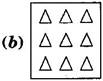(iii){tex}\frac{3}{5}{/tex} of the squares inbox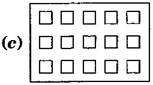(i) {tex}\frac{1}{2}{/tex} of 12 circles = {tex}\frac{1}{2} \times 12{/tex} = 6 circles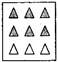(ii) {tex}\frac{2}{3}{/tex} of 9 triangles = {tex}\frac{2}{3} \times 9{/tex} = 2 x 3 = 6 triangles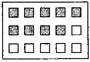(iii) {tex}\frac{3}{5}{/tex} of 15 squares = {tex}\frac{3}{5} \times 15{/tex} 3 x 3 = 9 squares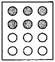NCERT Solutions for Class 7 Maths Exercise 2.2

###### Question 5.Find:

(a) {tex}\frac{1}{2}{/tex} of (i) 24 (ii) 46

(b) {tex}\frac{2}{3}{/tex} of (i) 18 (ii) 27

(c) {tex}\frac{3}{4}{/tex} of (i) 16 (ii) 36

(d) {tex}\frac{4}{5}{/tex} of (i) 20 (ii) 35

(a)

(i) {tex}\frac{1}{2}{/tex} of 24 = 12

(ii) {tex}\frac{1}{2}{/tex} of 46 = = 23

(b)

(i) {tex}\frac{2}{3}{/tex} of 18 = {tex}\frac{2}{3} \times 18{/tex} = 2 x 6 = 12

(ii) {tex}\frac{2}{3}{/tex} of 27 = {tex}\frac{2}{3} \times 27{/tex} = 2 x 9 = 18

(c)

(i) {tex}\frac{3}{4}{/tex} of 16 = {tex}\frac{3}{4} \times 16{/tex} = 3 x 4 = 12

(ii) {tex}\frac{3}{4}{/tex} of 36 = {tex}\frac{3}{4} \times 36{/tex} = 3 x 9 = 27

(d)

(i) {tex}\frac{4}{5}{/tex} of 20 = {tex}\frac{4}{5} \times 20{/tex} = 4 x 4 = 16

(ii) {tex}\frac{4}{5}{/tex} of 35 = {tex}\frac{4}{5} \times 35{/tex} = 4 x 7 = 28

NCERT Solutions for Class 7 Maths Exercise 2.2

###### Question 6.Multiply and express as a mixed fraction:

(a) {tex}3 \times 5\frac{1}{5}{/tex}

(b) {tex}5 \times 6\frac{3}{4}{/tex}

(c) {tex}7 \times 2\frac{1}{4}{/tex}

(d) {tex}4 \times 6\frac{1}{3}{/tex}

(e) {tex}3\frac{1}{4} \times 6{/tex}

(f) {tex}3\frac{2}{5} \times 8{/tex}

(a) {tex}3 \times 5\frac{1}{5} = 3 \times \frac{{26}}{5} = \frac{{3 \times 26}}{5} = \frac{{78}}{5} = 15\frac{3}{5}{/tex}

(b) {tex}5 \times 6\frac{3}{4} = 5 \times \frac{{27}}{4} = \frac{{5 \times 27}}{4} = \frac{{135}}{4} = 33\frac{3}{4}{/tex}

(c) {tex}7 \times 2\frac{1}{4} = 7 \times \frac{9}{4} = \frac{{7 \times 9}}{4} = \frac{{63}}{4} = 15\frac{3}{4}{/tex}

(d) {tex}4 \times 6\frac{1}{3} = 4 \times \frac{{19}}{3} = \frac{{4 \times 19}}{3} = \frac{{76}}{3} = 25\frac{1}{3}{/tex}

(e) {tex}3\frac{1}{4} \times 6 = \frac{{13}}{4} \times 6 = \frac{{13 \times 3}}{2} = \frac{{39}}{2} = 19\frac{1}{2}{/tex}

(f) {tex}3\frac{2}{5} \times 8 = \frac{{17}}{5} \times 8 = \frac{{17 \times 8}}{5} = \frac{{136}}{5} = 27\frac{1}{5}{/tex}

NCERT Solutions for Class 7 Maths Exercise 2.2

###### Question 7.Find:

(a) {tex}\frac{1}{2}{/tex} of (i) {tex}2\frac{3}{4}{/tex} (ii) {tex}4\frac{2}{9}{/tex}

(b) {tex}\frac{5}{8}{/tex} of (i) {tex}3\frac{5}{6}{/tex} (ii) {tex}9\frac{2}{3}{/tex}

Given: Total quantity of water in bottle = 5 litres

(i) Vidya consumed = {tex}\frac{2}{5}{/tex} of 5 litres = {tex}\frac{2}{5} \times 5{/tex} = 2 litres

Thus, Vidya drank 2 litres water from the bottle.

(ii) Pratap consumed = {tex}\left( {1 – \frac{2}{5}} \right){/tex} part of bottle

= {tex}\frac{{5 – 2}}{5} = \frac{3}{5}{/tex} part of bottle

Pratap consumed {tex}\frac{3}{5}{/tex} of 5 litres water = {tex}\frac{3}{5} \times 5{/tex} = 3 litres

Thus, Pratap drank {tex}\frac{3}{5}{/tex} part of the total quantity of water.

NCERT Solutions for Class 7 Maths Exercise 2.2

###### Question 8. Vidya and Pratap went for a picnic. Their mother gave them a water bottle that contained 5 litres of water. Vidya consumed {tex}\frac{2}{5}{/tex} of the water. Pratap consumed the remaining water.

(i)How much water did Vidya drink?

(ii)What fraction of the total quantity of water did Pratap drink?

Given: Total quantity of water in bottle = 5 litres

(i) Vidya consumed = {tex}\frac{2}{5}{/tex} of 5 litres = {tex}\frac{2}{5} \times 5{/tex} = 2 litres

Thus, Vidya drank 2 litres water from the bottle.

(ii) Pratap consumed = {tex}\left( {1 – \frac{2}{5}} \right){/tex} part of bottle

= {tex}\frac{{5 – 2}}{5} = \frac{3}{5}{/tex} part of bottle

Pratap consumed {tex}\frac{3}{5}{/tex} of 5 litres water = {tex}\frac{3}{5} \times 5{/tex} = 3 litres

Thus, Pratap drank {tex}\frac{3}{5}{/tex} part of the total quantity of water.

## NCERT Solutions for Class 7 Maths Exercise 2.2

NCERT Solutions Class 7 Maths PDF (Download) Free from myCBSEguide app and myCBSEguide website. Ncert solution class 7 Maths includes text book solutions from Class 7 Maths Book . NCERT Solutions for CBSE Class 7 Maths have total 15 chapters. 7 Maths NCERT Solutions in PDF for free Download on our website. Ncert Maths class 7 solutions PDF and Maths ncert class 7 PDF solutions with latest modifications and as per the latest CBSE syllabus are only available in myCBSEguide.

## CBSE app for Students

To download NCERT Solutions for Class 7 Maths, Social Science Computer Science, Home Science, Hindi English, Maths Science do check myCBSEguide app or website. myCBSEguide provides sample papers with solution, test papers for chapter-wise practice, NCERT solutions, NCERT Exemplar solutions, quick revision notes for ready reference, CBSE guess papers and CBSE important question papers. Sample Paper all are made available through the best app for CBSE students and myCBSEguide website.Test Generator

Create question paper PDF and online tests with your own name & logo in minutes.myCBSEguide

Question Bank, Mock Tests, Exam Papers, NCERT Solutions, Sample Papers, Notes

### 2 thoughts on “NCERT Solutions for Class 7 Maths Exercise 2.2”

1. It’s to nice app
l like it too

2. Nice